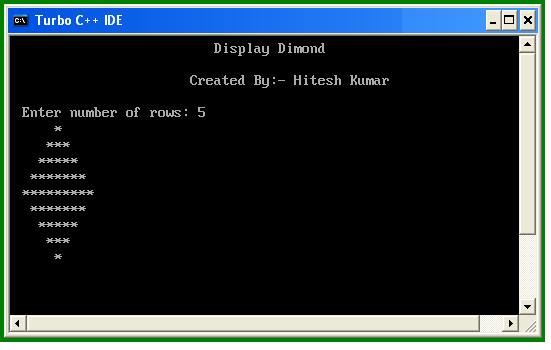# Print Diamond of Star in C

## Print Diamond of Stars in C

Using C language you can print diamond of stars, here you need to print two triangle, simply print first triangle and second triangle is reverse of first triangle.

## Print Diamond of Star in C

```#include<stdio.h>
#include<conio.h>

void main()
{
int n, c, k, space = 1;
clrscr();
printf("\n\nEnter number of rows: ");
scanf("%d", &n);
space = n - 1;

for (k = 1; k<=n; k++)
{
for (c = 1; c<=space; c++)
printf(" ");

space--;

for (c = 1; c<= 2*k-1; c++)
printf("*");

printf("\n");
}

space = 1;

for (k = 1; k<= n - 1; k++)
{
for (c = 1; c<= space; c++)
printf(" ");

space++;

for (c = 1 ; c<= 2*(n-k)-1; c++)
printf("*");

printf("\n");
}
getch();
}
```

OutputWIFI CCTV Camera